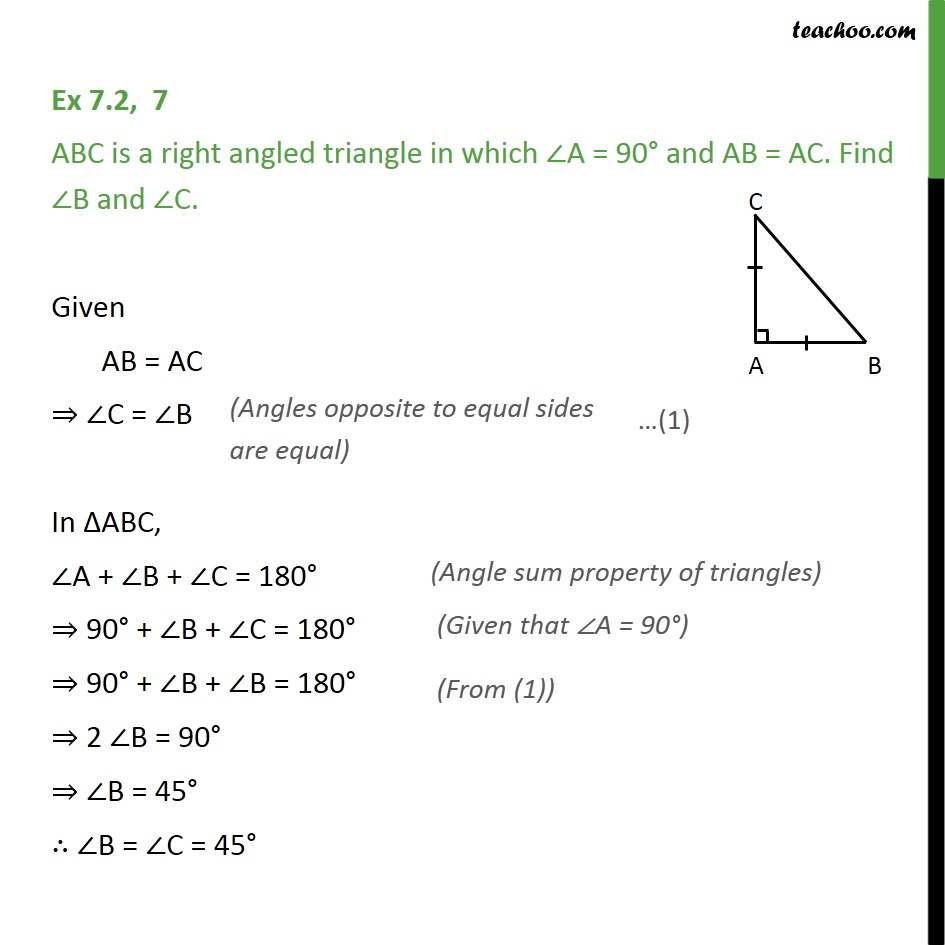1. Chapter 7 Class 9 Triangles (Term 1)
2. Serial order wise
3. Ex 7.2

Transcript

Ex 7.2, 7 ABC is a right angled triangle in which A = 90 and AB = AC. Find B and C. Given AB = AC C = B In ABC, A + B + C = 180 90 + B + C = 180 90 + B + B = 180 2 B = 90 B = 45 B = C = 45

Ex 7.2